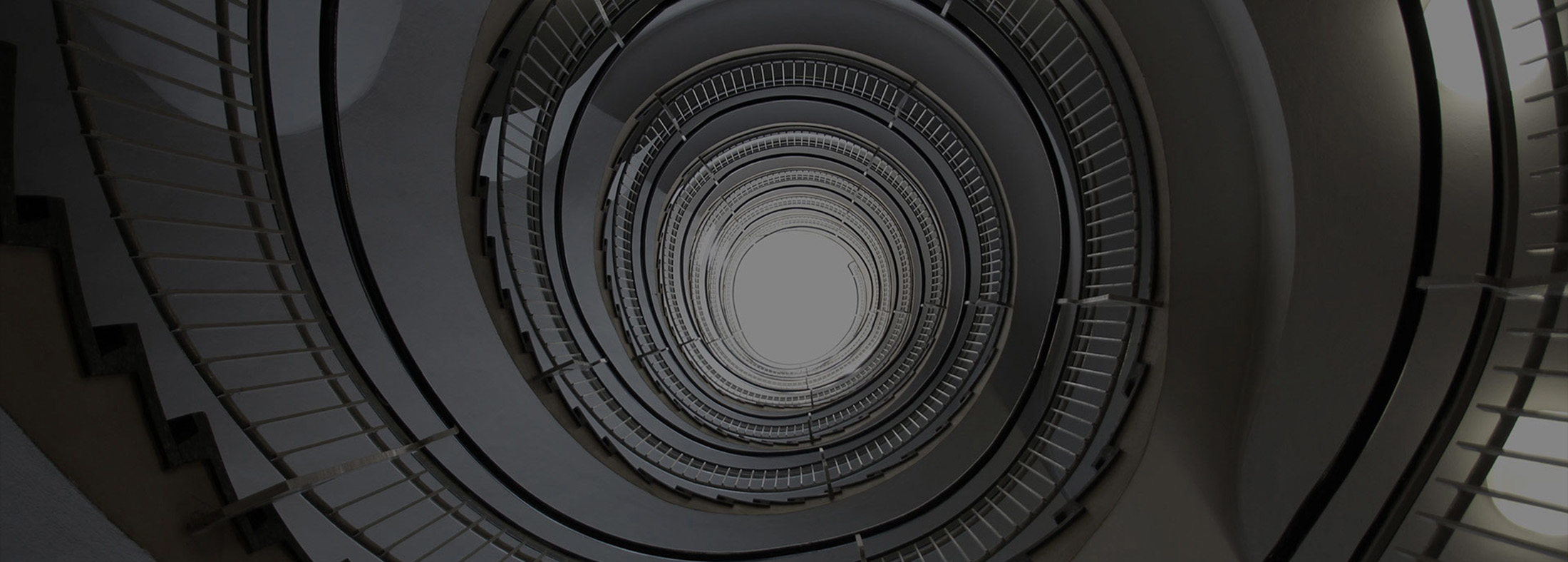# Secondary 1 Maths

##### Secondary School Maths

Our secondary maths tuition centre classes make concepts easy to understand and fun to apply.

## Our Lesson Syllabus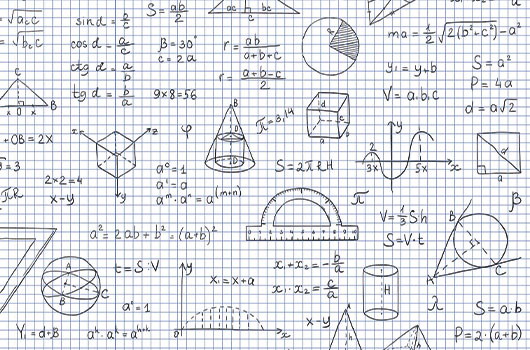### Term 1

1. Numbers and Operations

2. Prime Factor Decomposition

3. Introduction to Algebra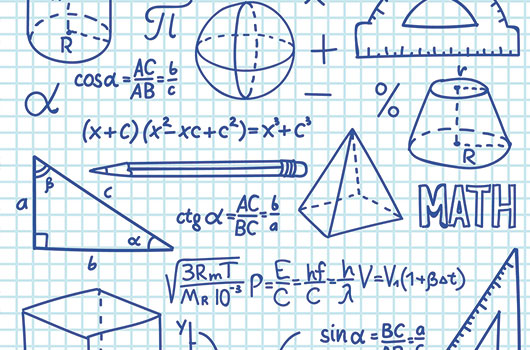### Term 2

1. Algebraic Fractions

2. Algebraic Equations

3. Applications of Algebra

4. Ratio, Proportion, Rate and Speed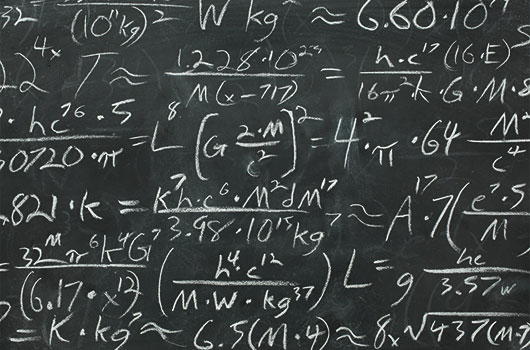### Term 3

1. Triangles and Geometry

2. Measuration

3. Linear Graphs

4. Statistics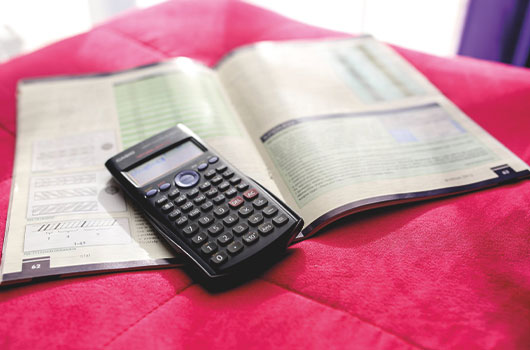### Term 4

1. Maps and Scales

2. Direct and Inverse Proportion

3. Simultaneous Equations

Need Help? Chat with us